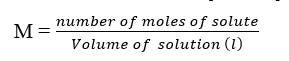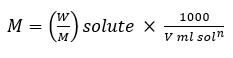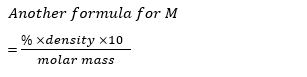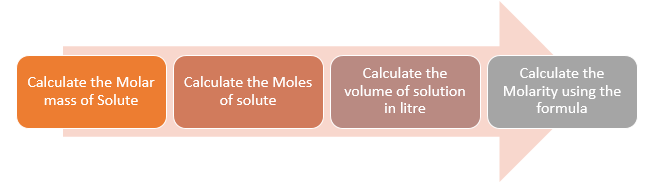# Molarity Formula, Definition, Molarity equation, Solved Examples

## Molarity Definition And Formula

It is quite widely used unit and it is denoted by letter M
It is the no. of moles of solute present in per litre solution. Unit = Moles per lit.
Molarity Formula is given asIf Volume = 1 litre
M = n solute
Unit of Molarity is Moles L-1
In terms of weight, molarity formula of the substance can be expressed as:Molarity formula with Density is given as## How to calculate the Molarity of a solution

1. First ,we need to calculate the Molar Mass of the solute. This can be find using the molar mass of the elements
Let's take a example of NaOH
Molar mass of NaOH = Molar mass of Na + Molar mass Of O + Molar mass of H
=23+16+1=40
2. We calculate the Moles of the solute by the formula
$n=\frac {\text{Mass(g)}}{\text{Molar Mass}}$
3. Find the Volume of Solution in Litre
We can use below table for our convenience
 1 cm3 1mL 1000 mm3 1 Litre 1000mL 1000 cm3 1 m3 106  cm3 1000 L 1 dm3 1000 cm3 1 L
4. Now Molarity can be simply calculated using the Formula of Molarity
$M= \frac {\text{No Of Moles of Solute}}{\text {Volume of Solution in (L)}}$## Solved Example of Molarity Formula

Question 1
Calculate the molarity of $NaOH$ in the solution prepared by dissolving its 4 g in enough water to form 250 mL of the solution.
Solution
As per Molarity Formula
$M = \frac {W}{M} \times \frac {1000}{\text{V ml}}$
= (4 g / 40 g ) ×  (1000/250) =0.1 mol/0.250 L
= 0.4 mol/L
= 0.4 M

Question 2
If 2 gm $NaOH$ is dissolute in water to make solution upto 250 cc. Give molarity of the solution:
Solution
NaOH =  W = 2 g
Molar Mass of NaOH = 23 + 16 + 1 = 40
M = W/M  ×  1000/(V ml)   = 2/40 × 1000/250=1/5 M

Question 3
Give the mass of NaOH dissolute to make solution of  500 cm3, 1/2 M molar.
Solution
M = W/M  ×  1000/(V ml)
Molar Mass of NaOH = 23 + 16 + 1 = 40
M=1/2  and V=500 ml
Therefore,
1/2=W/40  ×1000/500
W=20/2=10gm

Question 4
20% (w/W) NaOH solution (density of solution= 1.2 g/cc). Calculate the Molarity
Solution
20% (w/M) means 20 gm  in 100gm  of solution
Now Density of solution =1.2g/cc
So Volume of Solution =100/1.2 cc
Now
M = W/M  ×  1000/(V ml)
M= 20/40 ×  1000/100 × 1.2 = 6  M
Question 5
Give the volume of the solution if 10 gm NaOH, 1/20 M NaOH sol. is present.
Solution
M = W/M  ×  1000/(V ml)
1/20=10/40  ×  1000/(V ml)
4/20=  1000/(V ml)
V = 5000 ml
= 5L
Question 6
What is the amount of solute present in M/40,100 ml Na OH sol.
Solution
M = W/M  ×  1000/(V ml)
1/40=W/40  ×1000/100
4/40 = W
0.1 gm = W
Question 7
Calculate the morality of 2% (m/v) glucose sol.)
Solution
2 gm Glucose in 100 ml sol.
Now Molar mass of glucose(C6 H12 O6) = 12×6+1 ×12+6
= 72 + 12 + 96=180 gm

M = W/M  ×  1000/(V ml)
M = 2/180  ×1000/100
M = 1/9

## Molarity equation

To calculate the volume of a definite solution required to prepare solution of other molarity, the following equation is used:
M1 V1 = M2 V2
where M1= initial molarity
M2= molarity of the new solution
V1= initial volume
V2= volume of the new solution

Suppose we have mix three solution of same solute of Molarity M1 ,M2 ,M3 and Volume V1,V2 ,V3
Molarity of the resulting solution is given by
$M_R= \frac {M_1V_1 +M_2V_2 + M_3V_3}{V_1 + V_2 + V_3}$

## Solved Example of Molarity Equations

Question 1
If 500 mL of a 5M solution is diluted to 1500 mL, what will be the molarity of the solution obtained?
(i) 1.5 M
(ii) 1.66 M
(iii) 0.017 M
(iv) 1.59 M
Solution
M1 V1 = M2 V2
500 X 5=1500 M2
M2 =1.66 M
Question 2
If 4 g of NaOH dissolves in 36 g of H2O, calculate the mole fraction of each component in the solution. Also, determine the molarity of solution (specific
gravity of solution is 1g mL–1).
Solution
Mass of NaOH = 4 g
Number of moles of NaOH =4/40
= 0.1 mol

Mass of H2O = 36 g
Number of moles of  H2O= 36/18 =2 mol

Mole fraction of water
=Number of moles of H2O, /(No. of moles of water + No. of moles of NaOH)
=2/(2+.1)  = 0.95

Mole fraction of NaOH =Number of moles of NaOH/ (No. of moles of NaOH + No. of moles of water)

= .1 /(2+.1) = 0.047

Mass of solution = mass of water + mass of NaOH = 36 g + 4 g = 40 g
Volume of solution = 40 × 1 = 40 mL
(Since specific gravity of solution is = 1 g mL–1)
Molarity of solution
=Number of moles of solute/ Volume of solution in litre
=0.1 mol NaOH /.04 = 2.5 M
Question 3 How do I prepare 0.2 M HCL?
Solution
Now As per Molarity Formula
For 0.2M , we need 0.2 moles of HCl in 1 Litre Solution
Now Molecular mass of HCl=34.46 g/mol
1 mole of HCl contain $1 \times 34.46$ g HCl (no.of moles= given mass of solute/ molecular mass of solute)
0.2 moles of HCl contain $0.2 \times 34.46=6.892$g HCl
Therefore 6.892 g of HCl in a litre of solution gives 0.2 molar HCl solution

## Relation Between Mole Fraction and Molarity

Let χA = Mole fraction of solvent
χB = Mole Fraction of solute
nA = Number of moles of solvent
nB = Number of moles of solute
M = molarity of solution
d = Density of solution
MA = Molar mass of solvent
MB = Molar mass of solute

Now Mass of solution = nA MA + nBMB
Volume of solution = Mass of solution/density of solution
So, Volume of solution = (nA MA + nBMB)/d

The density of a solution is in g/mL

Molarity = Number of moles of Solute/ Volume of the solution
=nB × d × 1000/(nA MA + nBMB)
Dividing Numerator and denominator by nA+nB
= χB × d × 1000/(χA MA + χBMB)

If density is in g/litre then the molarity is given as
= χB × d/(χA MA + χBMB)
Check out Molarity Calculator

### Molarity Numerical Quiz

Question 1 Molarity of Solution having 2 moles of solutes present in 2 L of solution is
A. 1M
B. 2M
C. .5M
D. 4M
Question 2 What is the unit of Mole-fraction ?
A. it is unit less
B. moles/L
C. Moles/Kg
D. None of the above
Question 3 we mix three solution of same solute of Molarity 1M, 2M ,3M and Volume 1 L,2L ,3L. Find the molarity of the resultant solution
Molarity of the resulting solution is given by
A. 3.3M
B. 3M
C. 1M
D. 2.33M
Question 4 What is the molarity of a solution that contains 1.724 moles of solute in 2.50 L of solution?
A. 1.1M
B. .55M
C. 0.690 M
D. .670
Question 5 How many moles of NaCl are contained in 100.0 mL of a 0.200 M solution?
A. .002
B. 2
C. .02
D. .0002
Question 6find the molarity of the solution where mole fraction of solute is 0.5000 and Molar Mass of Solute is 49 g/Mole and Molar mass of solvent is 18 g/M and density of solution is 1.769 g/ml
A. 15.24
B. 11.13
C. 12.24
D. 10.1